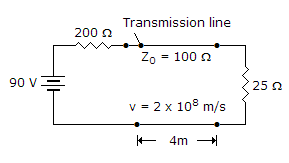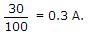# Electronics and Communication Engineering - Microwave Communication

### Exercise :: Microwave Communication - Section 4

6.

In a TWT the amplitude of resultant wave travelling down the helix remains constant

 A. True B. False

Explanation:

The signal gets strengthened.

7.

In the given figure forward current wave has a magnitude ofA. 0.45 A B. 0.3 A C. 0.2 A D. 0.1 A

Explanation:8.

In an infinite line the input impedance at every point is equal to characteristic impedance.

 A. True B. False

Explanation:

No answer description available for this question. Let us discuss.

9.

Consider the following time parameters

1. Domain growth time constant.
2. Transit time.
3. Dielectric relaxation time in positive mobility regime.
Which of the above are used in connection width transferred electron device?

 A. 1, 2, and 3 B. 1 and 2 only C. 1 and 3 only D. 2 and 3 only

Explanation:

No answer description available for this question. Let us discuss.

10.

PIN diode has

 A. p+ and n layers separated by i layer B. p+ and n+ layers separated by i layer C. p- and n- layers separated by i layer D. none of the above